Figure 5

### C = 2

Figure 10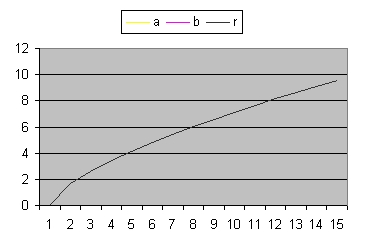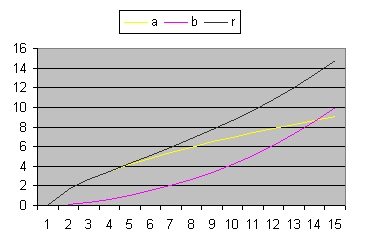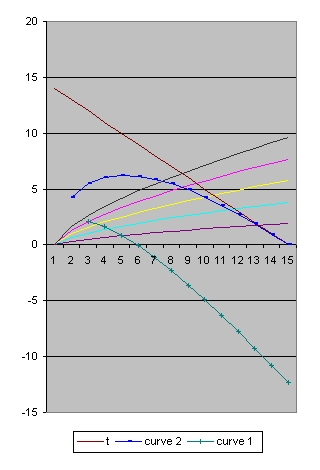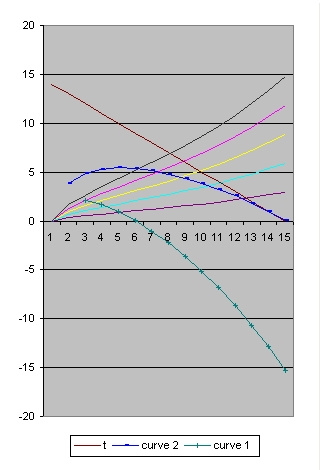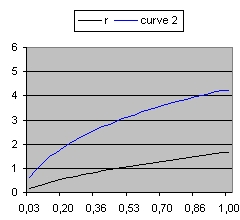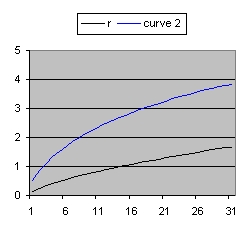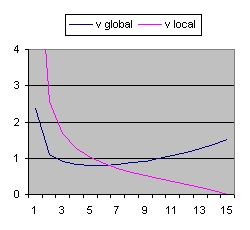Figure 5
C=2 Lambda=0
Omega(M) = 0.999952
Rho = 0.001082
Figure 10
C=2 Lambda=0.03
Omega(M) = 0.058214
Rho = 0.000295
When you compare the pictures from the left with the right than they are almost identical.
What that means is that based what is observed (redshift) it is very difficult to distinquish between the two.
Specific what is difficult is to calculate the cosmological constant. The main reason is because the influence locally is very small.

Back to Friedmann's Equation Question 1
Back to Friedmann's Equation Question 3